NCERT Solutions for Class 8 Maths Chapter 14 Factorisation Ex 14.1 are part of NCERT Solutions for Class 8 Maths. Here we have given NCERT Solutions for Class 8 Maths Chapter 14 Factorisation Ex 14.1.

 Board CBSE Textbook NCERT Class Class 8 Subject Maths Chapter Chapter 14 Chapter Name Factorisation Exercise Ex 14.1, Ex 14.2, Ex 14.3, Ex 14.4 Number of Questions Solved 3 Category NCERT Solutions

## NCERT Solutions for Class 8 Maths Chapter 14 Factorisation Ex 14.1

Question 1.
Find the common factors of the given terms:Solution.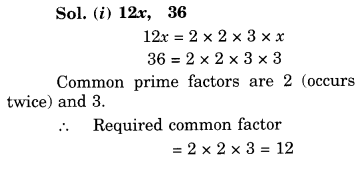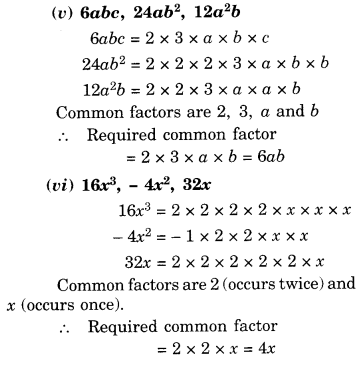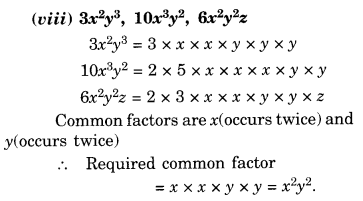Question 2.
Factorise the following expressions: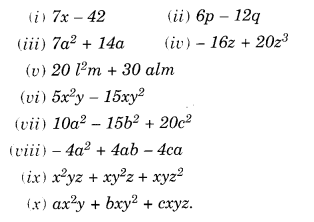Solution.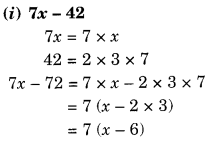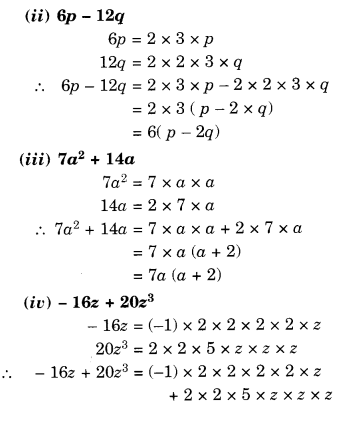Question 3.
Factorise: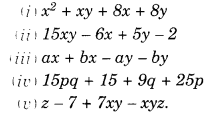Solution.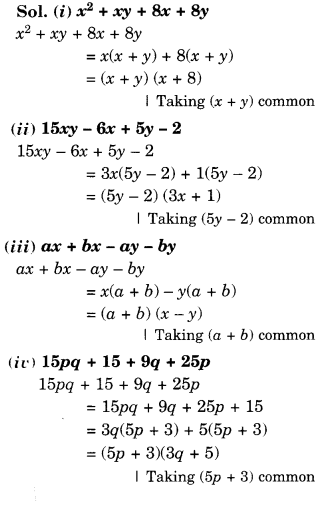We hope the NCERT Solutions for Class 8 Maths Chapter 14 Factorisation Ex 14.1 help you. If you have any query regarding NCERT Solutions for Class 8 Maths Chapter 14 Factorisation Ex 14.1, drop a comment below and we will get back to you at the earliest.